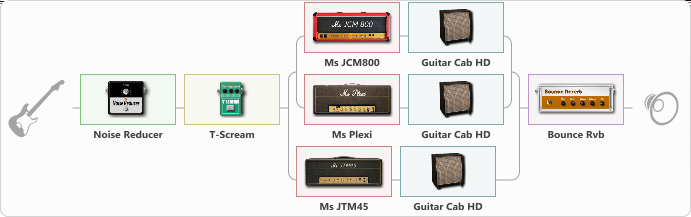# Ghost Opus Eponymous Album

Discussion in 'ToneLib-GFX presets' started by qmanworld, May 16, 2023.

1. Ghost Opus Eponymous Album

Preset name: Empty Preset

Effects chain:Effect: "Noise Reducer" (Dynamics / Filter), active - "yes"
{
"Sens" = 69
"Mode" = Soft
}

Effect: "T-Scream" (Overdrive / Distortion), active - "yes"
{
"Drive" = 22
"Tone" = 80
"Level" = 79
}

Effect: "Splitter" (Dynamics / Filter)
{
"A-Bypass" = Off
"A-Pan" = -25
"A-Level" = 55
"B-Bypass" = Off
"B-Pan" = 25
"B-Level" = 55
"Width" = 0

'A' branch:
{

Effect: "Splitter" (Dynamics / Filter)
{
"A-Bypass" = Off
"A-Pan" = -25
"A-Level" = 55
"B-Bypass" = Off
"B-Pan" = 25
"B-Level" = 55
"Width" = 0

'A' branch:
{

Effect: "Ms JCM800" (Amp simulators), active - "yes"
{
"Gain" = 62
"Bass" = 37
"Middle" = 50
"Treble" = 74
"Presence" = 50
"Master" = 89
"Level (dB)" = 0
}

Effect: "Guitar Cab HD" (Cabinets), active - "yes"
{
"Model" = Marshall 1960A (4x12")
"Mic Model" = Dynamic SM57
"Mic Position" = Middle
"Mic Distance" = Middle
"Low Cut (Hz)" = 45
"Hi Cut (kHz)" = 8.0
"Mix" = 100
"Level (dB)" = 0
}
}
'B' branch:
{

Effect: "Ms Plexi" (Amp simulators), active - "yes"
{
"Gain" = 100
"Bass" = 42
"Middle" = 50
"Treble" = 100
"Presence" = 50
"Master" = 77
"Level (dB)" = 0
}

Effect: "Guitar Cab HD" (Cabinets), active - "yes"
{
"Model" = Marshall 1960A (4x12")
"Mic Model" = Dynamic SM57
"Mic Position" = Middle
"Mic Distance" = Middle
"Low Cut (Hz)" = 45
"Hi Cut (kHz)" = 8.0
"Mix" = 100
"Level (dB)" = 0
}
}
}
}
'B' branch:
{

Effect: "Ms JTM45" (Amp simulators), active - "yes"
{
"Gain" = 69
"Bass" = 36
"Middle" = 50
"Treble" = 78
"Presence" = 59
"Master" = 52
"Level (dB)" = 0
}

Effect: "Guitar Cab HD" (Cabinets), active - "yes"
{
"Model" = Marshall 1960A (4x12")
"Mic Model" = Dynamic SM57
"Mic Position" = Middle
"Mic Distance" = Middle
"Low Cut (Hz)" = 45
"Hi Cut (kHz)" = 8.0
"Mix" = 100
"Level (dB)" = 0
}
}
}

Effect: "Bounce Rvb" (Reverberation), active - "yes"
{
"Time" = 3.9
"PreDelay" = 0
"LoDamp" = 16
"HiDamp" = 26
"Mix" = 42
}

Note: You will need to download and install the ToneLib-GFX software to use the preset.

#### Attached Files:

• ###### Ghost_Opus_Eponymous_Album.tlgfx
File size:
1.1 KB
Views:
3,841
VIDALO likes this.